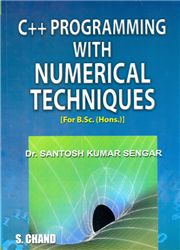# C++ Programming With Numerical Techniques, 1/e Dr. Santosh Kumar Sengar-S.CHAND

175.00 155.00

Introducing C++ | Solution Of Algebraic And Transcendental Equations | System Of Linear Algebraic Equations | Calculus Of Finitedifferences | Interpolation With Equal Intervals | Central Differenceinterpolation | Interpolation With Unequal Intervals | Numericaldifferentiation | Numerical Integration | Numerical Solution Ofordinary Differential Equations | Tables | Index